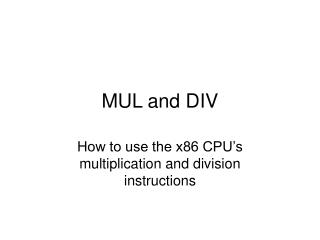DownloadDownload PresentationMUL and DIV

MUL and DIV

Télécharger la présentationMUL and DIV

- - - - - - - - - - - - - - - - - - - - - - - - - - - E N D - - - - - - - - - - - - - - - - - - - - - - - - - - -
Presentation Transcript

1. MUL and DIV How to use the x86 CPU’s multiplication and division instructions

2. A comment about addition • When two register-values are added add %al, %bl their total can sometimes be “too large” to fit in the destination register: Example: 150 + 160 = 310 • Both numbers 150 and 160 can be expressed as 8-bit binary values: 150 as 10010110 (base 2) 160 as 10100000 (base 2) • But not their sum: 310 is 100110110 (base 2)

3. The Carry-Flag • The “extra” bit becomes the CF-bit (i.e., the ‘Carry’ Flag) in the RFLAGS register • Programs can “branch” if a carry occurs: jc toobig • Or branch elsewhere if no-carry occurs: jnc sumok • Special instruction exists to handle adding of large numbers: ADC (Add-with-Carry)

4. A Big Number example .section .data x: .short 150 y: .short 160 .section .text mov x, %al add y, %al mov x+1, %ah adc y+1, %ah

5. “worst-cases” add vs multiply • Adding two n-bit numbers never requires more than (n+1)-bits for their total, so the carry-flag bit suffices for holding the result • But multiplication is another story! • Biggest 8-bit number is 255 (= 11111111) • 255x255 = 65025 (= 1111111000000001) • So this product requires 16-bits: 0xFE01

6. MUL • Using MUL to multiply two 64-bit operands • Put the 64-bit ‘multiplicand’ into RAX • Put the 64-bit ‘multiplier’ into a general register • The CPU will produce a 128-bit ‘product’ • The product will be in the (RDX:RAX) register-pair • (You can also put the 64-bit ‘multiplier’ in a memory-variable if you then use ‘MULQ’)

7. DIV • If you put your 128-bit ‘dividend’ into the RDX:RAX register-pair, then you can put your 64-bit divisor into a general-register • The ‘quotient’ will appear in RAX and the remainder will appear in RDX – unless the quotient is too large to fit in register RAX • (You can also put your 64-bit ‘divisor’ in a memory variable if you then use DIVQ)